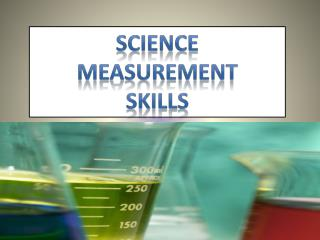Download PresentationScience Measurement skills

# Science Measurement skills

Download Presentation## Science Measurement skills

- - - - - - - - - - - - - - - - - - - - - - - - - - - E N D - - - - - - - - - - - - - - - - - - - - - - - - - - -
##### Presentation Transcript

1. Science Measurement skills

2. Metric System(System International or SI) • The metric system is used in science so that the entire world will be using the same system. • It is based on the number 10. • Units of measurement: • Mass = Gram (g) • Volume = Liters (L) • Length = Meter (m) • Density = Grams per milliliter (g/ml) • Temperature = Celsius (c) or Kelvin (k) • Force = Newtons (n)

3. Metric prefixes • Prefixes can be added to any unit whenever larger or smaller measurements are needed: • milli (m)- one one-thousandth; 0.001 • centi (c)- one one-hundredth; 0.01 • Deci (d)- one tenth; 0.1 • Base Unit (Ex.= Gram, Liter, Meter)– one; 1 • deka (da)- ten; 10 • hecto (h)- one hundred; 100 • kilo (k) - one thousand; 1,000 ... and a few you might want to know … • nano (n) = 1 one-billionth = 0.000000001 • micro (µ) - one one-millionth; 0.000001 • mega (M)- one million; 1,000,000 • giga (G)- one billion; 1,000,000,000

4. Metric Conversions • In order to convert larger or smaller metric units you must count the steps of the metric prefixes and then move the decimal point to the left when going down the steps and right when going up the steps: Step 1= Milli Step 2= centi Step 3= deci Step 4= Unit (m, L, g) Step 5= deka Step 6= hecto Step 7 = kilo Move decimal to the left Move decimal to the right

5. Examples: • You need to convert 2.5 Meters into centimeters. There are you take two steps up from meters to get to centimeters. 2.5000 M = 250.00 cm Step 1= Milli Step 2= centi Step 3= deci Step 4= Unit (M) Step 5= deka Step 6= hecto Step 7 kilo

6. Practice: • Change the following: • 25000 liters into Kiloliters • 450 millimeters into meters • 4,500,000 centigrams into Kilograms • 195 centimeters into meters • 5 Kilometers into meters • 15 Kiloliters into milliliters

7. Massbase unit is a gram (g) • Mass is the amount of matter in an object. It is measured in grams with a balance. • A triple beam balance uses known masses, and balances them with unknown masses. Can you tell what the mass of the object on this balance is?

8. Volume Base Unit is a Liter (l) • Volume is the measurement of how much space an object takes up, in other words its size. Volume is measured in liters with tools such as a graduated cylinder. Meniscus 142 ml

9. LengthBase Unit is a Meter (m) The base unit of length in the metric system is the meter. A meter is just a little longer than a yard (39.5 inches).

10. TemperatureUnit of measurement is Celsius • Temperature is measured in degrees Celsius in the metric system. The Celsius scale is based on the boiling and freezing points of water at sea level. Water freezes at 0° and boils at 100 °.

11. DensityMeasured in grams per milliliter (liquids) or grams per centimeters cubed (solids) • The amount of mass in a given volume is density. Elements are measured in grams per milliliter (or grams per centimeters cubed). Water has a density of 1 g/ml. If a substance has a higher density it sinks, and if it has a lower density it floats.

12. ForceBase Unit is a Newton (n) • Weight is a measurement of the pull of gravity (a force). on an objects mass The greater the gravitational pull the higher the weight. That is why a person weighs more on earth than they would on the moon. The metric unit for force is a newton. 1 newton is 0.22 pounds. A spring scale is used to measured an objects weight.